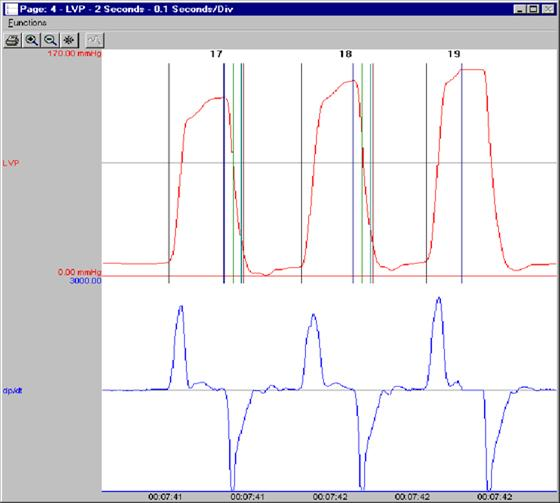# Left Ventricular Pressure Analysis Module

The Left Ventricular Pressure (LVP) Analysis Module computes physiologically meaningful parameters from digitized left ventricular pressure data.  The graph above represents a typical left ventricular pressure recording and its first derivative (dP/dt) as they would appear on the monitor.  Automated validation marks for Left Ventricular End Diastolic Pressure, Systolic Pressure, Percent Recovery Times and -dP/dt max are shown (additional validation marks are available).  The validation marks provide visual, real-time verification of the accuracy of the system.

The list below describes the parameters calculated by the analysis module either in real-time or during subsequent analysis.

##### Model PNM-LVP100W
 Name Definition Num The number of the cardiac cycle. Sys The systolic pressure is the maximum pressure that occurs during the cardiac cycle. LVEDP The left ventricular end diastolic pressure is the pressure at the last zero crossing of the differentiated pressure during the rise to the systolic period. EMw Electromechanical Window represents the time difference in milliseconds between the end of electrical systole (end of the T wave) and the end of ventricular relaxation. It is a potential biomarker for Torsades de Pointes (TdP) risk that has greater predicability than using QT prolongation. Min The minimum pressure during the cardiac cycle. TTI Tension-Time Index is the area under the left ventricular pressure during the ejection phase of the contraction. This is the integration between the LVEDP point and -dP/dtMAX. DP Developed pressure is the difference between the systolic pressure and the left ventricular end diastolic pressure (SYS-LVEDP). HR The heart rate is computed in beats-per-minute. It is calculated by taking the reciprocal of the time interval for the cardiac cycle multiplied by 60. +dP/dt +dP/dt is the maximum positive value of the first derivative of the pressure that occurs during the cardiac cycle. -dP/dt -dP/dt is the maximum negative value of the first derivative of the pressure that occurs during the cardiac cycle. CI Contractility index is +dP/dt divided by the pressure at that point. RT1, RT2 The Relaxation Time is the time period from -dP/dt to the time specified by the Relaxation Time attribute. The time is reported in milliseconds. dP (A, B, C, and D) These parameters report the value of dP/dt at the pressure levels specified in dP/dt A, dP/dt B, dP/dt C, and dP/dt D (in the attributes window). NPMN The non-pulsatile mean pressure reported for a logging period. This parameter is still reported even if no pulse pressure exists. Q-A The Q-A Interval is the time in milliseconds from the start of the Q-wave, in the ECG trigger channel, to the start of the systolic pressure rise (LVEDP). IVT The time in milliseconds from the start of the systolic pressure rise (LVEDP) to the maximum slope of the systolic pressure rise (+dP/dt). TTI-T LVEDP to -dP/dt. The time is in milliseconds. Tau Tau is the time constant isovolumic left ventricular pressure decay. It is reported in milliseconds, and can be defined using Pressure, Developed Pressure and dP/dt. Period The duration of the current cycle time, in milliseconds. Count Reports the number of cycles in a given logging period.